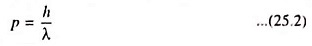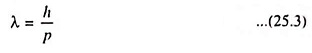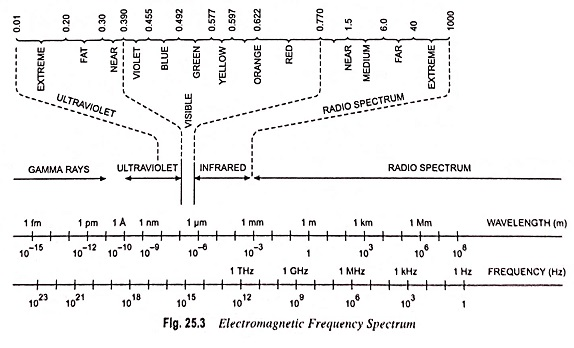## What is Wave Particle Duality?

Since waves exhibit particle-like behavior, then the particles also should exhibit wave—like properties. This theory (de Broglie) is based on the existence of a wave particle duality principle. The momentum of a photon is given aswhere λ is the wavelength of the light wave.

Then, de Broglie hypothesized that the wavelength of a particle can be expressed aswhere p is the momentum of the particle and λ is known as the de Broglie wavelength of the matter wave. Typical wavelengths used for such experiments are in ultraviolet and visible range, these being very short compared to the usual radio spectrum range. Figure 25.3 shows the electromagnetic frequency spectrum.Figure 25.4 shows the light spectrum plotted against wavelength and energy. This figure includes the relative response of the human eye.

Scroll to Top